# 动量弹球交易策略

15 一月 2018, 08:40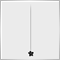0
8 264

### 动量弹球交易策略的规则

L. Raschke 和 L. Connors 在使用由 George Taylor 描述的交易技巧时遇到了不确定的问题，这也是他们编写这个交易策略规则的原因。在泰勒的策略中，在一天之前会确定它交易的方向 - 这一天将是卖出的一天或是买入的一天。但是，作者实际的交易经常会违反这一决定，根据书作者的观点，这会使交易规则变得混乱，

1. 在 D1 时段中, LBR/RSI 在最新关闭的日柱上应该在超卖区域之内 - 低于 30.
2. 在新的一天中的第一个小时柱关闭之后，在高于那个柱的最高点处设置买入挂单。
3. 在挂单被触发之后，把止损设置到第一个小时柱的最低价。
4. 如果仓位因为止损而关闭，再次在同一水平设置卖出挂单。
5. 如果在当天结束之前，仓位还是获利的，就把它留到下一天。在第二个交易日，仓位必须关闭。

— LBR/RSI 在每日时段处于超卖区域 (参见2017年10月30日)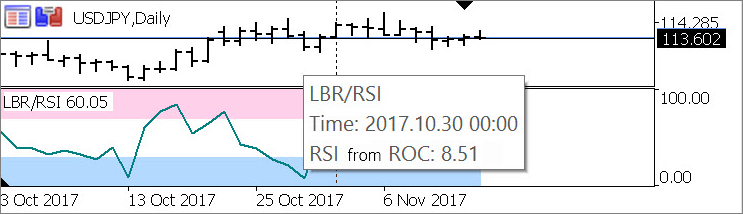— TS_Momentum_Pinball 位于不确定的时段 (从 M1 到 D1) 显示了交易水平和这一天第一个小时的价格范围, 这些水平就根据它们来计算的: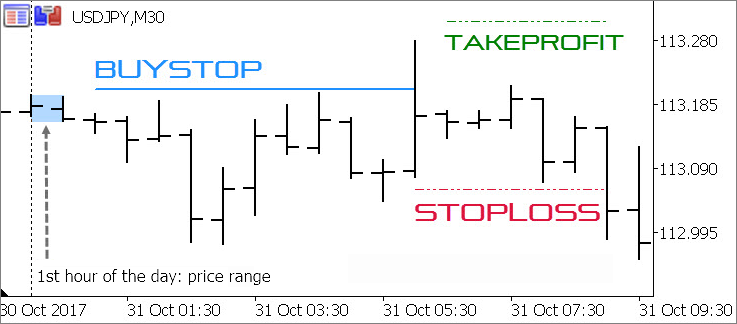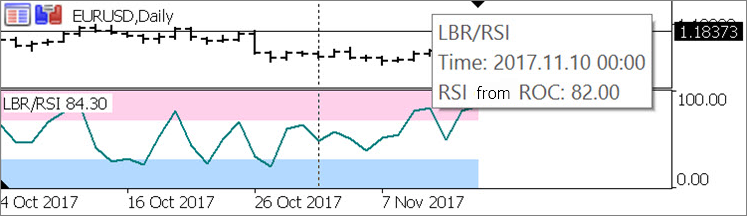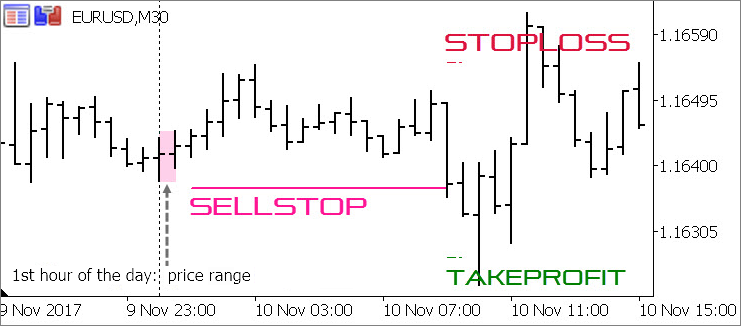### LBR/RSI 指标

```#property indicator_separate_window
#property indicator_buffers  9
#property indicator_plots    3
#property indicator_label1  “超买区域"
#property indicator_type1   DRAW_FILLING
#property indicator_color1  C'255,208,234'
#property indicator_width1  1
#property indicator_label2  “超卖区域"
#property indicator_type2   DRAW_FILLING
#property indicator_color2  C'179,217,255'
#property indicator_width2  1
#property indicator_label3  "RSI от ROC"
#property indicator_type3   DRAW_LINE
#property indicator_style3  STYLE_SOLID
#property indicator_color3  clrTeal
#property indicator_width3  2
#property indicator_minimum 0
#property indicator_maximum 100
input ENUM_APPLIED_PRICE  TS_MomPin_Applied_Price = PRICE_CLOSE;  // 用于 ROC 计算的价格
input uint    TS_MomPin_RSI_Period = 3;                           // RSI 周期数
input double  TS_MomPin_RSI_Overbought = 70;                      // RSI 超卖水平
input double  TS_MomPin_RSI_Oversold = 30;                        // RSI 超买水平
double
buff_Overbought_High[], buff_Overbought_Low[],                  // 超买区域背景
buff_Oversold_High[], buff_Oversold_Low[],                      // 超卖区域背景
buff_Price[],                                                   // 计算价格的数组
buff_ROC[],                                                     // 来自计算价格的 ROC 数组
buff_RSI[],                                                     // ROC 的 RSI
buff_Positive[], buff_Negative[]                                // RSI 计算的辅助数组
;
int OnInit() {
// 指标缓冲区设计:

// 超买区域
SetIndexBuffer(0, buff_Overbought_High, INDICATOR_DATA);
PlotIndexSetDouble(0, PLOT_EMPTY_VALUE, EMPTY_VALUE);
PlotIndexSetInteger(0, PLOT_SHOW_DATA, false);
SetIndexBuffer(1, buff_Overbought_Low, INDICATOR_DATA);

// 超卖区域
SetIndexBuffer(2, buff_Oversold_High, INDICATOR_DATA);
PlotIndexSetDouble(1, PLOT_EMPTY_VALUE, EMPTY_VALUE);
PlotIndexSetInteger(1, PLOT_SHOW_DATA, false);
SetIndexBuffer(3, buff_Oversold_Low, INDICATOR_DATA);

// RSI 曲线
SetIndexBuffer(4, buff_RSI, INDICATOR_DATA);
PlotIndexSetDouble(2, PLOT_EMPTY_VALUE, EMPTY_VALUE);

// RSI 计算使用的缓冲区
SetIndexBuffer(5, buff_Price, INDICATOR_CALCULATIONS);
SetIndexBuffer(6, buff_ROC, INDICATOR_CALCULATIONS);
SetIndexBuffer(7, buff_Negative, INDICATOR_CALCULATIONS);
SetIndexBuffer(8, buff_Positive, INDICATOR_CALCULATIONS);

IndicatorSetInteger(INDICATOR_DIGITS, 2);
IndicatorSetString(INDICATOR_SHORTNAME, "LBR/RSI");

return(INIT_SUCCEEDED);
}
```

```int
i_RSI_Period = int(TS_MomPin_RSI_Period),         // 把 RSI 周期数转换为整数类型
i_Bar, i_Period_Bar                               // 用于同时访问的两个柱的索引
;
double
d_Sum_Negative, d_Sum_Positive,                   // 用于 RSI 计算的辅助变量
d_Change                                          // 用于 ROC 计算的辅助变量
;
// Fill in ROC buffer and fill areas:
i_Period_Bar = 1;
while(++i_Period_Bar < rates_total && !IsStopped()) {
// 计算的柱的价格:
switch(TS_MomPin_Applied_Price) {
case PRICE_CLOSE:     buff_Price[i_Period_Bar] = Close[i_Period_Bar]; break;
case PRICE_OPEN:      buff_Price[i_Period_Bar] = Open[i_Period_Bar]; break;
case PRICE_HIGH:      buff_Price[i_Period_Bar] = High[i_Period_Bar]; break;
case PRICE_LOW:       buff_Price[i_Period_Bar] = Low[i_Period_Bar]; break;
case PRICE_MEDIAN:    buff_Price[i_Period_Bar] = 0.50000 * (High[i_Period_Bar] + Low[i_Period_Bar]); break;
case PRICE_TYPICAL:   buff_Price[i_Period_Bar] = 0.33333 * (High[i_Period_Bar] + Low[i_Period_Bar] + Open[i_Period_Bar]); break;
case PRICE_WEIGHTED:  buff_Price[i_Period_Bar] = 0.25000 * (High[i_Period_Bar] + Low[i_Period_Bar] + Open[i_Period_Bar] + Open[i_Period_Bar]); break;
}
// 计算价格的差 (ROC 数值):
if(i_Period_Bar > 1) buff_ROC[i_Period_Bar] = buff_Price[i_Period_Bar] - buff_Price[i_Period_Bar - 2];

// 填充背景:
buff_Overbought_High[i_Period_Bar] = 100;
buff_Overbought_Low[i_Period_Bar] = TS_MomPin_RSI_Overbought;
buff_Oversold_High[i_Period_Bar] = TS_MomPin_RSI_Oversold;
buff_Oversold_Low[i_Period_Bar] = 0;
}
```

```i_Period_Bar = prev_calculated - 1;
if(i_Period_Bar <= i_RSI_Period) {
buff_RSI = buff_Positive = buff_Negative = d_Sum_Positive = d_Sum_Negative = 0;
i_Bar = 0;
while(i_Bar++ < i_RSI_Period) {
buff_RSI = buff_Positive = buff_Negative = 0;
d_Change = buff_ROC[i_Bar] - buff_ROC[i_Bar - 1];
d_Sum_Positive += (d_Change > 0 ? d_Change : 0);
d_Sum_Negative += (d_Change < 0 ? -d_Change : 0);
}
buff_Positive[i_RSI_Period] = d_Sum_Positive / i_RSI_Period;
buff_Negative[i_RSI_Period] = d_Sum_Negative / i_RSI_Period;

if(buff_Negative[i_RSI_Period] != 0)
buff_RSI[i_RSI_Period] = 100 - (100 / (1. + buff_Positive[i_RSI_Period] / buff_Negative[i_RSI_Period]));
else
buff_RSI[i_RSI_Period] = buff_Positive[i_RSI_Period] != 0 ? 100 : 50;

i_Period_Bar = i_RSI_Period + 1;
}

i_Bar = i_Period_Bar - 1;
while(++i_Bar < rates_total && !IsStopped()) {
d_Change = buff_ROC[i_Bar] - buff_ROC[i_Bar - 1];

buff_Positive[i_Bar] = (buff_Positive[i_Bar - 1] * (i_RSI_Period - 1) + (d_Change> 0 ? d_Change : 0)) / i_RSI_Period;
buff_Negative[i_Bar] = (buff_Negative[i_Bar - 1] * (i_RSI_Period - 1) + (d_Change <0 ? -d_Change : 0)) / i_RSI_Period;

if(buff_Negative[i_Bar] != 0)
buff_RSI[i_Bar] = 100 - 100. / (1. + buff_Positive[i_Bar] / buff_Negative[i_Bar]);
else
buff_RSI[i_Bar] = buff_Positive[i_Bar] != 0 ? 100 : 50;
}```

### 信号模块

• 使用跟踪止损来平仓;
• 在第二天早晨平仓;
• 等到第二天突破建仓日价格极值的时候平仓。

“早晨” 是一个不确定的概念，为了使规则更加规范，需要更确切的定义。Raschke 和 Connors 没有谈到它，但是假如把24小时时间尺度中的'早晨'绑定为第一个日柱(在另外的交易策略规则中都是如此使用的)，这也是比较合理的。

```enum ENUM_EXIT_MODE {     // 退场方法列表
CLOSE_ON_SL_TRAIL,      // 只通过跟踪止损
CLOSE_ON_NEW_1ST_CLOSE, // 通过在后面一天的第一个柱平仓
CLOSE_ON_DAY_BREAK      // 通过突破建仓日价格极值平仓
};
// user settings
input ENUM_APPLIED_PRICE  TS_MomPin_Applied_Price = PRICE_CLOSE;     // 动量弹球: 用于计算 ROC 的价格
input uint    TS_MomPin_RSI_Period = 3;                              // 动量弹球: RSI 周期数
input double  TS_MomPin_RSI_Overbought = 70;                         // 动量弹球: RSI 超卖水平
input double  TS_MomPin_RSI_Oversold = 30;                           // 动量弹球: RSI 超买水平
input uint    TS_MomPin_Entry_Offset = 10;                           // 动量弹球: 入场水平距离 H1 边界的偏移(点数)
input uint    TS_MomPin_Exit_Offset = 10;                            // 动量弹球: 出场水平距离 H1 边界的偏移(点数)
input ENUM_EXIT_MODE  TS_MomPin_Exit_Mode = CLOSE_ON_SL_TRAIL;       // 动量弹球: 获利仓位的平仓方法
```

```ENUM_ENTRY_SIGNAL fe_Get_Entry_Signal(      // 两个柱形模式分析 (D1 + H1)
datetime  t_Time,                         // 当前时间
double&    d_Entry_Level,                 // 进场水平 (与变量的连接)
double&    d_SL,                          // 止损水平 (与变量的连接)
double&    d_TP,                          // 获利水平 (与变量的连接)
double&    d_Range_High,                  // 范围内第一个小时柱的最高价 (与变量的连接)
double&    d_Range_Low                    // 范围内第一个小时柱的最低价 (与变量的连接)
) {
// function body
}
```

```static ENUM_ENTRY_SIGNAL se_Trade_Direction = ENTRY_UNKNOWN;   // 今天的交易方向
static double
// 用于在分时之间保存计算的水平的变量
sd_Entry_Level = 0,
sd_SL = 0, sd_TP = 0,
sd_Range_High = 0, sd_Range_Low = 0
;
if(t_Time < 0) {                                               // 只用于在指标中调用
sd_Entry_Level = sd_SL = sd_TP = sd_Range_High = sd_Range_Low = 0;
}
// 默认使用之前保存的水平来做进场/出场:
d_Entry_Level = sd_Entry_Level; d_SL = sd_SL; d_TP = sd_TP; d_Range_High = sd_Range_High; d_Range_Low = sd_Range_Low;
```

```static int si_Indicator_Handle = INVALID_HANDLE;
if(si_Indicator_Handle == INVALID_HANDLE) {
// 在第一次调用函数时取得指标句柄:
si_Indicator_Handle = iCustom(_Symbol, PERIOD_D1, "LBR_RSI",
TS_MomPin_Applied_Price,
TS_MomPin_RSI_Period,
TS_MomPin_RSI_Overbought,
TS_MomPin_RSI_Oversold
);

if(si_Indicator_Handle == INVALID_HANDLE) { // 没有得到指标句柄
if(Log_Level > LOG_LEVEL_NONE) PrintFormat("%s: 收取 LBR_RSI 指标句柄出错代码 #%u", __FUNCTION__, _LastError);
return(ENTRY_INTERNAL_ERROR);
}
}
```

```static int si_Indicator_Handle = INVALID_HANDLE;
if(si_Indicator_Handle == INVALID_HANDLE) {
// receiving indicator handle at first function call:
si_Indicator_Handle = iCustom(_Symbol, PERIOD_D1, "LBR_RSI",
TS_MomPin_Applied_Price,
TS_MomPin_RSI_Period,
TS_MomPin_RSI_Overbought,
TS_MomPin_RSI_Oversold
);

if(si_Indicator_Handle == INVALID_HANDLE) {       // 没有取得指标句柄
if(Log_Level > LOG_LEVEL_NONE) PrintFormat("%s: 指标句柄接收错误 LBR_RSI #%u", __FUNCTION__, _LastError);
return(ENTRY_INTERNAL_ERROR);
}
}
// 查找前一天日柱的时间:
datetime ta_Bar_Time[];
if(CopyTime(_Symbol, PERIOD_D1, fabs(t_Time), 2, ta_Bar_Time) < 2) {
if(Log_Level > LOG_LEVEL_NONE) PrintFormat("%s: CopyTime: 错误 #%u", __FUNCTION__, _LastError);
return(ENTRY_INTERNAL_ERROR);
}
// 如果这是今天第一次调用，对前一天的分析:
static datetime st_Prev_Day = 0;
if(t_Time < 0) st_Prev_Day = 0;                     // only for call from indicator
if(st_Prev_Day < ta_Bar_Time) {
// 之前一天参数清零:
d_Entry_Level = sd_Entry_Level = d_SL = sd_SL = d_TP = sd_TP = d_Range_High = sd_Range_High = d_Range_Low = sd_Range_Low = 0;

// 取得前一天的 LBR/RSI 指标值:
double da_Indicator_Value[];
if(1 > CopyBuffer(si_Indicator_Handle, 4, ta_Bar_Time, 1, da_Indicator_Value)) {
if(Log_Level > LOG_LEVEL_NONE) PrintFormat("%s: CopyBuffer: 错误 #%u", __FUNCTION__, _LastError);
return(ENTRY_INTERNAL_ERROR);
}

// 如果 LBR/RSI 指标值有错误:
if(da_Indicator_Value > 100. || da_Indicator_Value < 0.) {
if(Log_Level > LOG_LEVEL_NONE) PrintFormat("%s: 指标缓冲区值出错 (%f)", __FUNCTION__, da_Indicator_Value);
return(ENTRY_UNKNOWN);
}

st_Prev_Day = ta_Bar_Time;                     // 重试次数计数

// 记录今天的交易方向:
if(da_Indicator_Value > TS_MomPin_RSI_Overbought) se_Trade_Direction = ENTRY_SELL;

// 记到记录中:
if(Log_Level == LOG_LEVEL_DEBUG) PrintFormat("%s: 交易方向 %s: %s. LBR/RSI: (%.2f)",
__FUNCTION__,
TimeToString(ta_Bar_Time, TIME_DATE),
da_Indicator_Value
);
}
```

```// 今天没有搜索信号
// 分析今天第一个H1上的小时柱，除非已经做过了:
if(sd_Entry_Level == 0.) {
// to receive data of last 24 bars H1:
MqlRates oa_H1_Rates[];
int i_Price_Bars = CopyRates(_Symbol, PERIOD_H1, fabs(t_Time), 24, oa_H1_Rates);
if(i_Price_Bars == WRONG_VALUE) {                      // 处理 CopyRates 函数错误
if(Log_Level > LOG_LEVEL_NONE) PrintFormat("%s: CopyRates: 错误 #%u", __FUNCTION__, _LastError);
return(ENTRY_INTERNAL_ERROR);
}

// 在24个柱中找到今天的第一个柱，并且记录最高价和最低价:
int i_Bar = i_Price_Bars;
while(i_Bar-- > 0) {
if(oa_H1_Rates[i_Bar].time < ta_Bar_Time) break;      // 前一天在 H1 上的最后一个柱

// H1第一个柱的范围边界:
sd_Range_High = d_Range_High = oa_H1_Rates[i_Bar].high;
sd_Range_Low = d_Range_Low = oa_H1_Rates[i_Bar].low;
}
// H1 第一个柱还没有关闭:
if(i_Price_Bars - i_Bar < 3) return(ENTRY_UNKNOWN);

// 计算交易水平:

// 进入市场的水平:
d_Entry_Level = _Point * TS_MomPin_Entry_Offset;           // 辅助计算
sd_Entry_Level = d_Entry_Level = se_Trade_Direction == ENTRY_SELL ?d_Range_Low - d_Entry_Level : d_Range_High + d_Entry_Level;
// 初始止损水平:
d_SL = _Point * TS_MomPin_Exit_Offset;                     // 辅助计算
sd_SL = d_SL = se_Trade_Direction == ENTRY_BUY ?d_Range_Low - d_SL : d_Range_High + d_SL;
}
```

```ENUM_EXIT_SIGNAL fe_Get_Exit_Signal(    // 侦测平仓信号
double            d_Entry_Level,      // 进场水平
datetime          t_Entry_Time,       // 进场时间
datetime          t_Current_Time,     // 当前时间
ENUM_EXIT_MODE    e_Exit_Mode         // 退出模式
) {
static MqlRates soa_Prev_D1_Rate[];   // 前一天D1柱上的数据
static int si_Price_Bars = 0;         // 辅助计数器
if(t_Current_Time < 0) {              // 用于区分从指标中或是从EA中调用
t_Current_Time = -t_Current_Time;
si_Price_Bars = 0;
}
double
d_Curr_Entry_Level,
d_SL, d_TP,
d_Range_High,  d_Range_Low
;

if(e_Trade_Direction < 1) {          // 没有仓位，一切清零
si_Price_Bars = 0;
}

switch(e_Exit_Mode) {
case CLOSE_ON_SL_TRAIL:            // 只有跟踪止损
return(EXIT_NONE);

case CLOSE_ON_NEW_1ST_CLOSE:       // 在后面一天第一个柱时平仓
if((t_Current_Time - t_Current_Time % 86400)
==
(t_Entry_Time - t_Current_Time % 86400)
) return(EXIT_NONE);       // 建仓日尚未结束

if(fe_Get_Entry_Signal(t_Current_Time, d_Curr_Entry_Level, d_SL, d_TP, d_Range_High, d_Range_Low)
< ENTRY_UNKNOWN
) {
if(Log_Level > LOG_LEVEL_ERR) PrintFormat("%s: 后一天的第一个柱关闭了", __FUNCTION__);
return(EXIT_ALL);
}
return(EXIT_NONE);         // 不做平仓

case CLOSE_ON_DAY_BREAK:           // 根据突破建仓日价格极值平仓
if((t_Current_Time - t_Current_Time % 86400)
==
(t_Entry_Time - t_Current_Time % 86400)
) return(EXIT_NONE);       // 建仓日尚未结束

if(t_Current_Time % 86400 > 36000) return(EXIT_ALL); // 时间超出

if(si_Price_Bars < 1) {
si_Price_Bars = CopyRates(_Symbol, PERIOD_D1, t_Current_Time, 2, soa_Prev_D1_Rate);
if(si_Price_Bars == WRONG_VALUE) { // 处理 CopyRates 函数错误
if(Log_Level > LOG_LEVEL_NONE) PrintFormat("%s: CopyRates: 错误 #%u", __FUNCTION__, _LastError);
return(EXIT_UNKNOWN);
}

if(soa_Prev_D1_Rate.high < soa_Prev_D1_Rate.high) return(EXIT_NONE);        // 没有突破

if(Log_Level > LOG_LEVEL_ERR) PrintFormat("%s: 价格突破了昨天的最高价: %s > %s", __FUNCTION__, DoubleToString(soa_Prev_D1_Rate.high, _Digits), DoubleToString(soa_Prev_D1_Rate.high, _Digits));
} else {
if(soa_Prev_D1_Rate.low > soa_Prev_D1_Rate.low) return(EXIT_NONE);          // 没有突破

if(Log_Level > LOG_LEVEL_ERR) PrintFormat("%s: 价格突破了昨天的最低价: %s < %s", __FUNCTION__, DoubleToString(soa_Prev_D1_Rate.low, _Digits), DoubleToString(soa_Prev_D1_Rate.low, _Digits));
return(EXIT_SELL);
}
}

return(EXIT_NONE); // for each
}

return(EXIT_UNKNOWN);
}
```

```enum ENUM_EXIT_SIGNAL {  // 出场信号的列表
EXIT_UNKNOWN,          // 没有标记
EXIT_SELL,             // 关闭卖出仓位
EXIT_ALL,              // 全部平仓
EXIT_NONE              // 不做平仓
};
```

### 用于人工交易的指标

```#property indicator_chart_window
#property indicator_buffers  6
#property indicator_plots    4
#property indicator_label1  “一天中的第一个小时"
#property indicator_type1   DRAW_FILLING
#property indicator_color1  C'255,208,234', C'179,217,255'
#property indicator_width1  1
#property indicator_label2  “进场水平"
#property indicator_type2   DRAW_COLOR_LINE
#property indicator_style2  STYLE_DASHDOT
#property indicator_color2  clrDodgerBlue, clrDeepPink
#property indicator_width2  2
#property indicator_label3  "止损"
#property indicator_type3   DRAW_LINE
#property indicator_style3  STYLE_DASHDOTDOT
#property indicator_color3  clrCrimson
#property indicator_width3  1
#property indicator_label4  "获利"
#property indicator_type4   DRAW_LINE
#property indicator_color4  clrGreen
#property indicator_width4  1
```

```enum ENUM_LOG_LEVEL {  // 记录的级别
LOG_LEVEL_NONE,      // 禁止记录
LOG_LEVEL_ERR,       // 只记录错误
LOG_LEVEL_INFO,      // 记录错误和EA注释
LOG_LEVEL_DEBUG      // 全部记录，没有例外
};
enum ENUM_ENTRY_SIGNAL {  // 入场信号列表
ENTRY_SELL,             // 卖出信号
ENTRY_NONE,             // 没有信号
ENTRY_UNKNOWN,          // 状态不确定
ENTRY_INTERNAL_ERROR    // 内部函数错误
};
enum ENUM_EXIT_SIGNAL {  // 退出信号列表
EXIT_UNKNOWN,          // 没有识别
EXIT_SELL,             // 关闭卖出仓位
EXIT_ALL,              // 关闭全部
EXIT_NONE              // 不做关闭
};
#include <Expert\Signal\Signal_Momentum_Pinball.mqh>     // 动量弹球交易策略的信号模块
input uint    TS_MomPin_Take_Profit = 10;                // 动量弹球: 获利 (点数)
input bool    Show_1st_H1_Bar = true;                    // 是否显示每天第一个小时柱的范围?
input bool    Alert_Popup = true;                        // 提醒: 显示弹出窗口?
input bool    Alert_Email = false;                       // 提醒: 发送 e-mail?
input string  Alert_Email_Subj = "";                     // 提醒: e-mail 提醒的主题
input bool    Alert_Push = true;                         // 提醒: 发送推送通知?
input uint  Days_Limit = 7;                              // 历史深度 (日历天数)
ENUM_LOG_LEVEL  Log_Level = LOG_LEVEL_DEBUG;             // 记录模式
double
buff_1st_H1_Bar[], buff_1st_H1_Bar_Zero[],             // 用于填充每天第一个小时柱的缓冲区
buff_Entry[], buff_Entry_Color[],                      // 挂单线的缓冲区
buff_SL[],                                             // 止损线的缓冲区
buff_TP[],                                             // 获利线的缓冲区
gd_Entry_Offset = 0,                                   // 交易品种价格的 TS_MomPin_Entry_Offset
gd_Exit_Offset = 0                                     // 交易品种价格的 TS_MomPin_Exit_Offset
;
```

```int OnInit() {
// 把点数转换为交易品种的价格:
gd_Entry_Offset = TS_MomPin_Entry_Offset * _Point;
gd_Exit_Offset = TS_MomPin_Exit_Offset * _Point;

// 指标缓冲区的设计:

// 每日第一个小时柱的长方形
SetIndexBuffer(0, buff_1st_H1_Bar, INDICATOR_DATA);
PlotIndexSetDouble(0, PLOT_EMPTY_VALUE, 0);
SetIndexBuffer(1, buff_1st_H1_Bar_Zero, INDICATOR_DATA);
PlotIndexSetDouble(1, PLOT_EMPTY_VALUE, 0);

// 挂单设置线
SetIndexBuffer(2, buff_Entry, INDICATOR_DATA);
PlotIndexSetDouble(1, PLOT_EMPTY_VALUE, 0);
SetIndexBuffer(3, buff_Entry_Color, INDICATOR_COLOR_INDEX);

// 止损线
SetIndexBuffer(4, buff_SL, INDICATOR_DATA);
PlotIndexSetDouble(2, PLOT_EMPTY_VALUE, 0);

// 获利线
SetIndexBuffer(5, buff_TP, INDICATOR_DATA);
PlotIndexSetDouble(3, PLOT_EMPTY_VALUE, 0);

IndicatorSetInteger(INDICATOR_DIGITS, _Digits);
IndicatorSetString(INDICATOR_SHORTNAME, "Momentum Pinball");

return(INIT_SUCCEEDED);
}
```

```go_Brownie.f_Update(prev_calculated, prev_calculated);     // “提供” 数据给 Brownie
datetime t_Time = TimeCurrent();                           // 最近知道的服务器时间
int
i_Period_Bar = 0,                                        // 辅助计数器
i_Current_TF_Bar = 0                                     // 循环起始柱的索引
;
if(go_Brownie.b_First_Run) {                               // 如果这是第一次运行
i_Current_TF_Bar = rates_total — Bars(_Symbol, PERIOD_CURRENT, t_Time — t_Time % 86400 — 86400 * Days_Limit, t_Time);
// clearing buffer at re-initialization:
ArrayInitialize(buff_1st_H1_Bar, 0); ArrayInitialize(buff_1st_H1_Bar_Zero, 0);
ArrayInitialize(buff_Entry, 0); ArrayInitialize(buff_Entry_Color, 0);
ArrayInitialize(buff_TP, 0);
ArrayInitialize(buff_SL, 0);
} else if(!go_Brownie.b_Is_New_Bar) return(rates_total);   // 等待柱的关闭
else {                                                     // 新柱
// 最小重新计算深度 - 从开始的一天计算:
i_Current_TF_Bar = rates_total — Bars(_Symbol, PERIOD_CURRENT, t_Time — t_Time % 86400, t_Time);
}
ENUM_ENTRY_SIGNAL e_Entry_Signal = ENTRY_UNKNOWN;          // 入场信号
double
d_SL = WRONG_VALUE,                                      // 止损水平
d_TP = WRONG_VALUE,                                      // 获利水平
d_Entry_Level = WRONG_VALUE,                             // 入场水平
d_Range_High = WRONG_VALUE, d_Range_Low = WRONG_VALUE    // 模式的第一个柱的范围边界
;
datetime
t_Curr_D1_Bar = 0,                                       // 当前D1柱的时间 (模式的第二个柱)
t_Last_D1_Bar = 0,                                       // 最近的D1柱的时间，信号可以在这个柱上
t_Entry_Bar = 0                                          // 设置挂单的柱的时间
;
// 确保初始重计算柱的索引在允许的范围之内:
i_Current_TF_Bar = int(fmax(0, fmin(i_Current_TF_Bar, rates_total — 1)));
```

```while(++i_Current_TF_Bar < rates_total && !IsStopped()) {                // 迭代当前时段的柱
// 从信号模块接收数据:
e_Entry_Signal = fe_Get_Entry_Signal(-Time[i_Current_TF_Bar], d_Entry_Level, d_SL, d_TP, d_Range_High, d_Range_Low);
if(e_Entry_Signal == ENTRY_INTERNAL_ERROR) {                           // 从外部指标缓冲区复制数据出错
// 计算和画图应当在下一个分时重复:
go_Brownie.f_Reset();
return(rates_total);
}
if(e_Entry_Signal > 1) continue;                                       // 这个柱上没有活动信号
```

t_Curr_D1_Bar = Time[i_Current_TF_Bar] - Time[i_Current_TF_Bar] % 86400; // 这个柱属于当天的起始时间

```t_Curr_D1_Bar = Time[i_Current_TF_Bar] - Time[i_Current_TF_Bar] % 86400;            // 柱所属于的当天的起始时间
if(t_Last_D1_Bar < t_Curr_D1_Bar) {                                                 // 这是有信号的当天的第一个柱
t_Entry_Bar = Time[i_Current_TF_Bar];                                             // 记住交易开始的时间
```

```// 填充第一个小时柱的背景:
if(Show_1st_H1_Bar) {
i_Period_Bar = i_Current_TF_Bar;
while(Time[--i_Period_Bar] >= t_Curr_D1_Bar && i_Period_Bar > 0)
if(e_Entry_Signal == ENTRY_BUY) {                               // 牛市模式

buff_1st_H1_Bar_Zero[i_Period_Bar] = d_Range_High;
buff_1st_H1_Bar[i_Period_Bar] = d_Range_Low;
} else {                                                        // 熊市模式
buff_1st_H1_Bar[i_Period_Bar] = d_Range_High;
buff_1st_H1_Bar_Zero[i_Period_Bar] = d_Range_Low;
}
}
```

```// 入场线，直到与柱相交叉:
i_Period_Bar = i_Current_TF_Bar - 1;
if(e_Entry_Signal == ENTRY_BUY) {                               // 牛市模式
while(++i_Period_Bar < rates_total) {
if(Time[i_Period_Bar] > t_Curr_D1_Bar + 86399) {            // 一天的结束
e_Entry_Signal = ENTRY_NONE;                              // 挂单没有被触发
break;
}

// 延长线:
buff_Entry[i_Period_Bar] = d_Entry_Level;
buff_Entry_Color[i_Period_Bar] = 0;

if(d_Entry_Level <= High[i_Period_Bar]) break;               // 在这个柱有入场
}
} else {                                                         // 熊市模式
while(++i_Period_Bar < rates_total) {
if(Time[i_Period_Bar] > t_Curr_D1_Bar + 86399) {             // 一天结束
e_Entry_Signal = ENTRY_NONE;                               // 挂单没有触发
break;
}

// 延长线:
buff_Entry[i_Period_Bar] = d_Entry_Level;
buff_Entry_Color[i_Period_Bar] = 1;

if(d_Entry_Level >= Low[i_Period_Bar]) break;               // 在这个柱有入场
}
}
```

```if(e_Entry_Signal == ENTRY_NONE) {                 // 挂单在一天结束之前没有触发
i_Current_TF_Bar = i_Period_Bar;                 // 我们对这一天的柱就不再感兴趣
continue;
}
```

if(i_Period_Bar >= rates_total - 1) break;        // 当前 (还未结束) 日完成了

```// 挂单被触发，查找仓位关闭的柱:
i_Period_Bar = fmin(i_Period_Bar, rates_total - 1);
buff_SL[i_Period_Bar] = d_SL;
while(++i_Period_Bar < rates_total) {
if(TS_MomPin_Exit_Mode == CLOSE_ON_SL_TRAIL) {
if(Time[i_Period_Bar] >= t_Curr_D1_Bar + 86400) break;        // 这是后一天的柱

// 画获利和止损线，直到它们与价格柱有交叉:
buff_SL[i_Period_Bar] = d_SL;
buff_TP[i_Period_Bar] = d_TP;

if((
e_Entry_Signal == ENTRY_BUY && d_SL >= Low[i_Period_Bar]
) || (
e_Entry_Signal == ENTRY_SELL && d_SL <= High[i_Period_Bar]
)) {                                                          // 止损退出
if(buff_SL[int(fmax(0, i_Period_Bar - 1))] == 0.) {
// 在一个柱上开始和结束，向过去延伸一个柱
buff_SL[int(fmax(0, i_Period_Bar - 1))] = d_SL;
buff_TP[int(fmax(0, i_Period_Bar - 1))] = d_TP;
}
break;
}

if((
e_Entry_Signal == ENTRY_BUY && d_TP <= High[i_Period_Bar]
) || (
e_Entry_Signal == ENTRY_SELL && d_SL >= Low[i_Period_Bar]
)) {                                                         // 获利退出
if(buff_TP[int(fmax(0, i_Period_Bar - 1))] == 0.) {
// 在一个柱上开始和结束，向过去延伸一个柱
buff_SL[int(fmax(0, i_Period_Bar - 1))] = d_SL;
buff_TP[int(fmax(0, i_Period_Bar - 1))] = d_TP;
}
break;
}
}
}
```

```i_Period_Bar = i_Current_TF_Bar;
t_Curr_D1_Bar = Time[i_Period_Bar] - Time[i_Period_Bar] % 86400;
while(
++i_Period_Bar < rates_total
&&
t_Curr_D1_Bar == Time[i_Period_Bar] - Time[i_Period_Bar] % 86400
) i_Current_TF_Bar = i_Period_Bar;
```

```i_Period_Bar = rates_total - 1;                                            // 当前柱
if(t_Entry_Bar != Time[i_Period_Bar]) return(rates_total);                 // 这个柱没有信号
// message wording:
string s_Message = StringFormat("ТС Momentum Pinball: 需要 %s @ %s, SL: %s",
DoubleToString(d_Entry_Level, _Digits),
DoubleToString(d_SL, _Digits)
);
// 提醒:
```

### 用于测试动量弹珠交易策略的 EA 交易

```} else {                       // 不是新的仓位
// 用于关闭仓位的条件准备好了吗?
ENUM_EXIT_SIGNAL e_Exit_Signal = fe_Get_Exit_Signal(d_Entry_Level, datetime(PositionGetInteger(POSITION_TIME)), e_Entry_Signal, TimeCurrent(), TS_MomPin_Exit_Mode);
if((
) || (
e_Exit_Signal == EXIT_SELL && e_Entry_Signal == ENTRY_SELL
) || e_Exit_Signal == EXIT_ALL
) {
// 它必须被关闭
return;
}
}
```

### 策略的回测

EA交易使用的是从 MetaQuotes 模拟服务器2014年开始的报价，测试的余额变化图表。交易品种 — EURJPY, 时段 — H1: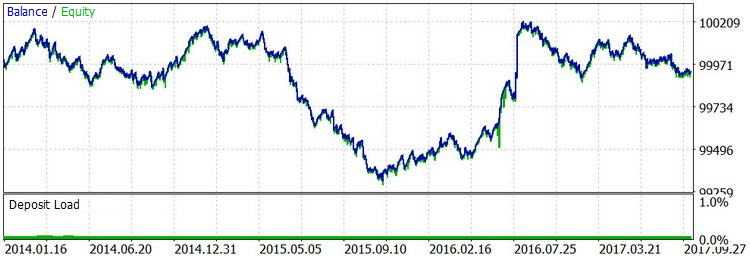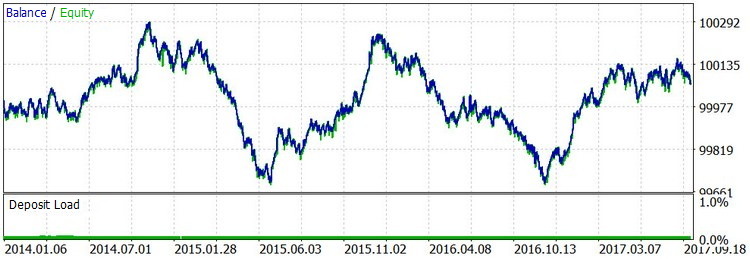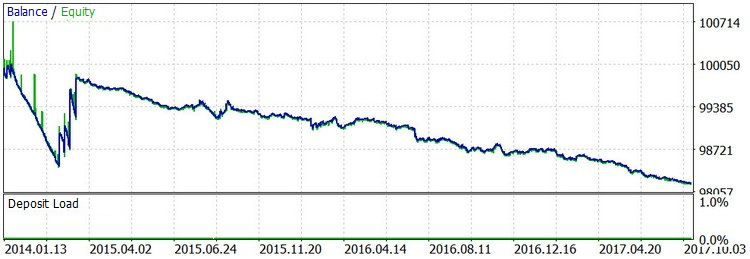### 结论

# File name 类型  描述
1  LBR_RSI.mq5  指标 合并了ROC和RSI的指标. 用于确定一天开始交易的方向(或者它的禁止状态)
2  TS_Momentum_Pinball.mq5  指标 使用本交易策略人工交易的指标。显示了计算的入场和出场水平，突出显示了开始计算时第一个小时的范围
3   Signal_Momentum_Pinball.mqh  开发库  函数，结构和用户设置的开发库，用于指标和EA交易中
4  Street_Smarts_Bot_MomPin.mq5   EA 交易 使用本交易策落进行自动交易的EA

MPtest.zip (744.43 KB)
MQL5.zip (163.27 KB)

#### 该作者的其他文章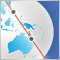在亚洲市场进行夜间交易: 如何保持盈利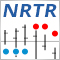用于 MQL5 向导的 NRTR 指标和交易模块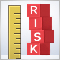单一资产交易顺序中的风险评估. 续篇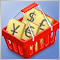测试当交易货币对篮子时出现的形态第二部分